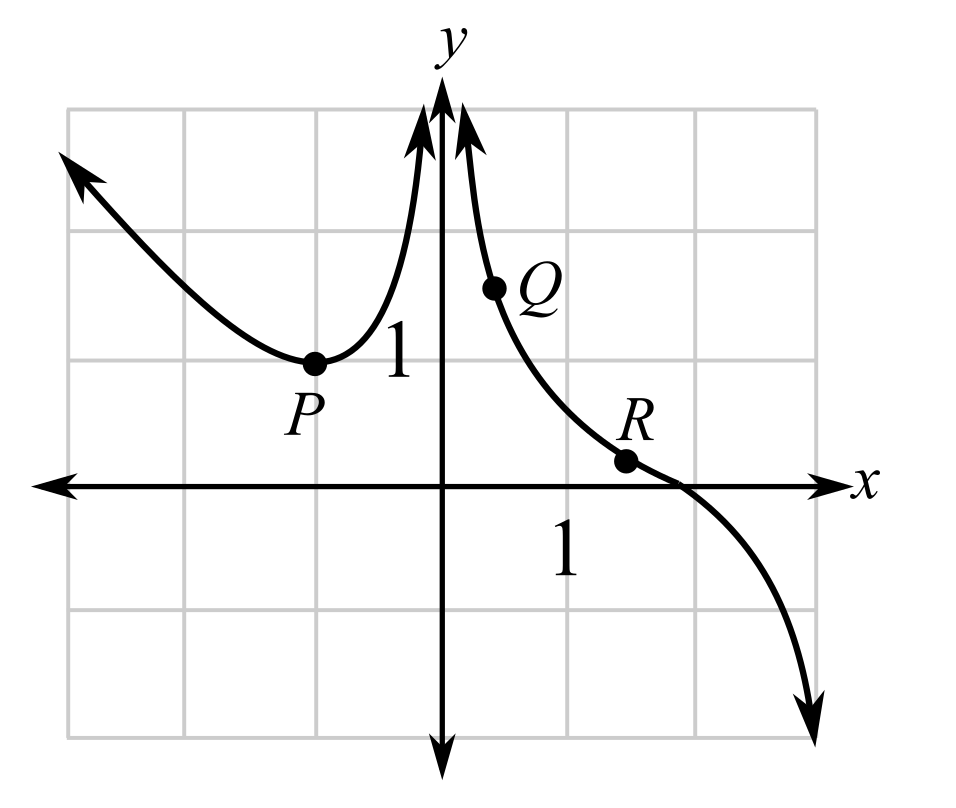Chapter 10.5, Problem 22EFinite Mathematics and Applied Cal...

7th Edition
Stefan Waner + 1 other
ISBN: 9781337274203

Solutions

Chapter
SectionFinite Mathematics and Applied Cal...

7th Edition
Stefan Waner + 1 other
ISBN: 9781337274203
Textbook Problem

In Exercises 17–22, say at which labeled point the slope of the tangent is (a) greatest and (b) least (in the sense that − 7 is less than 1). [HINT: See Quick Example 3.](a)

To determine

The greatest slope of the tangent among the labeled points in the graph which is as follows:Explanation

Given information:

The provided graph is:

When a function is increasing or rising the slope of the function is greatest where the tangent is more slanted whereas when the function is decreasing or falling it has the least value where the tangent is the most slanted.

From the graph one can observe that the function is first decreasing up to P then, increasing and then is discontinuous and then decreasing...

(b)

To determine

The least slope of the tangent among the labeled points in the graph which is as follows:Still sussing out bartleby?

Check out a sample textbook solution.

See a sample solution

The Solution to Your Study Problems

Bartleby provides explanations to thousands of textbook problems written by our experts, many with advanced degrees!

Get Started

Evaluate the indefinite integral. e5rdr

Single Variable Calculus: Early Transcendentals, Volume I

Evaluate Evaluate the expression. 15. 2422

Precalculus: Mathematics for Calculus (Standalone Book)

CHECK POINT 6. Simplify.

Mathematical Applications for the Management, Life, and Social Sciences